HP Forums

Full Version: [VA] SRC #009 - Pi Day 2021 Special
You're currently viewing a stripped down version of our content. View the full version with proper formatting.
Pages: 1 2 3
(03-15-2021 08:59 PM)J-F Garnier Wrote: [ -> ]I don't know -and don't think there is - any relation that can be used to get $$\pi$$ from e.

Et pourtant, il y en a – and yet there is at least one:

http://oeis.org/wiki/A_remarkable_formula_of_Ramanujan

Really remarkable, isn’t it?

${\int_{-\infty}^{ \infty}\rm{e}^{-{{x}}^{2}}\rm{d}x}=\sqrt{\pi}$
(03-16-2021 08:28 PM)robve Wrote: [ -> ]
I never posted "every programmer should write their own integration procedure". as the quotation suggests. What post are you referring to?

This one.  I quote:

"Also, what is the fun of doing math and calc exercises if we don't implement numerical integration ourselves?"

Regards.
V.
(03-16-2021 07:17 PM)Albert Chan Wrote: [ -> ]
(03-14-2021 07:00 PM)Valentin Albillo Wrote: [ -> ] [*]d.  Conversely, the volume enclosed by the n-dimensional sphere of radius R is given by: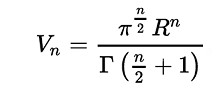Go on and evaluate the $$\pi$$-th root of the summation for even dimensions from 0 to $$\infty$$ of the volumes enclosed by the respective n-dimensional unit spheres (R = 1).

sum = 1 + pi/1! + pi²/2! + pi³/3! + ... = e^pi
sum ^ (1/pi) = e

Comment: formula give 1 for volume of 0-dimensional sphere, which seems weird.

Yes, it is kind of weird. But this is connecting two seemingly unrelated formulae, which is nice.

1. Taylor series:

$$e^x = 1+\frac{x}{1!}+\frac{x^2}{2!}+\frac{x^3}{3!}+\cdots$$

2. The volume of an n-ball with radius R:

$$V_n = \frac{\pi^{\frac{n}{2}}}{\Gamma(\frac{n}{2}+1)}R^n$$

The latter simplifies to

$$\frac{\pi^k}{k!}$$

for k=2n and R=1 (the conditions stated in the challenge). Therefore, the answer is e as the π root of the sum:

$$\sum_{k=0}^\infty \frac{\pi^k}{k!} = \mathrm{e}^\pi$$

I recognized the Taylor series after simplifying the sum's terms. Whenever you see a factorial in a denominator in a term of a series sum, there may be a Taylor series lurking beneath.

Nice piece of natural pie...

- Rob
(03-16-2021 09:09 PM)robve Wrote: [ -> ]$$\arctan u - \arctan v = \arctan\frac{u-v}{1+uv}$$

This is not quite right, LHS has possible range of ±pi, RHS is limited to ±pi/2
Correct identity is more complicated: see Sum of ArcTangents

We could use this instead: atan(u) ± atan(v) = atan2(u±v , 1∓uv) ﻿ ﻿ ﻿ ﻿ ﻿ ﻿ (*)

y = atan(x) - atan((x-1)/(x+1)) ﻿ ﻿ ﻿ ﻿ ﻿ ﻿ ﻿ ﻿ ﻿ ﻿ ﻿ ﻿ ﻿ ﻿ ﻿ ﻿ ﻿ ﻿ ﻿ ﻿ ﻿ ﻿ // y undefined when x = -1
﻿= atan2(x - (x-1)/(x+1), 1 + x*(x-1)/(x+1))
= atan2((x²+1)/(x+1), (x²+1)/(x+1)) ﻿ ﻿ ﻿ ﻿ ﻿ ﻿ ﻿ ﻿ ﻿ ﻿ ﻿ ﻿ // numerator always positive.

If x > -1, 4*y = 4*atan(1) = pi
If x < -1, 4*y = 4*(atan(1) - pi) = -3*pi

(*) Proof is trivial: (1+u*i) * (1±v*i) = (1∓u*v) + (u±v)*i

Phase angle of two sides matched, and we have above atan2 identity
(03-17-2021 02:32 AM)Albert Chan Wrote: [ -> ]
(03-16-2021 09:09 PM)robve Wrote: [ -> ]$$\arctan u - \arctan v = \arctan\frac{u-v}{1+uv}$$

This is not quite right, LHS has possible range of ±pi, RHS is limited to ±pi/2

Right. I did not include the two necessary conditions since these hold, note the (mod π):

$$\arctan u - \arctan v = \arctan\frac{u-v}{1+uv}\quad \pmod \pi,\quad uv\neq 1$$

EDIT: the arctan identity comes from

$$\tan \alpha \pm \tan \beta = \frac{\tan \alpha \pm \tan \beta}{1\mp \tan \alpha\, \tan \beta}$$

Since 0<=e<π and 0<=(e-1)/(e+1)<π we have (e.g. verify numerically)

$$\tan\arctan\mathrm{e}=\mathrm{e}; \quad \tan\arctan\frac{\mathrm{e}-1}{\mathrm{e}+1} = \frac{\mathrm{e}-1}{\mathrm{e}+1}$$

Then

$$\alpha=\arctan\mathrm{e};\quad \beta=\arctan \frac{\mathrm{e}-1}{\mathrm{e}+1};\quad \frac{\tan \alpha - \tan \beta}{1+ \tan \alpha\, \tan \beta} = \frac{\mathrm{e} - \frac{\mathrm{e}-1}{\mathrm{e}+1}}{1+\mathrm{e}\frac{\mathrm{e}-1}{\mathrm{e}+1}} = 1$$

Generally, tan has period π

$$\tan(k\pi+\theta) = \theta$$

for any integer k.

- Rob
(03-17-2021 12:49 AM)Valentin Albillo Wrote: [ -> ]
(03-16-2021 08:28 PM)robve Wrote: [ -> ]
I never posted "every programmer should write their own integration procedure". as the quotation suggests. What post are you referring to?

This one.  I quote:

"Also, what is the fun of doing math and calc exercises if we don't implement numerical integration ourselves?"

"Me/ourselves" and "implement integration" therefore "all programmers (should) write integration"?

As in "Socrates is a man, Socrates is mortal, therefore all men are mortal"?

Quotations matter.

Not a programmer, a D in Ph'y after passing some BS, humbly not wanting to be a P in the A, just taking some late snacks on vintage stuff to honor those that came before us.

- Rob
(03-16-2021 10:29 PM)Gerson W. Barbosa Wrote: [ -> ]
(03-15-2021 08:59 PM)J-F Garnier Wrote: [ -> ]I don't know -and don't think there is - any relation that can be used to get $$\pi$$ from e.

Et pourtant, il y en a – and yet there is at least one:

http://oeis.org/wiki/A_remarkable_formula_of_Ramanujan

Really remarkable, isn’t it?

${\int_{-\infty}^{ \infty}\rm{e}^{-{{x}}^{2}}\rm{d}x}=\sqrt{\pi}$

OK, I see what you (and Valentin probably too) mean and I agree of course.
By relation, I was (wrongly) limiting myself to finite expressions, like the arctan expression in Valentin's post. There are obviously many infinite sums and integrals involving e and producing pi in a way or another.

J-F
(03-14-2021 07:00 PM)Valentin Albillo Wrote: [ -> ][*]e.   $$\pi$$ also features in a song by Kate Bush (included in her 2005's album "Aerial") about a man who's utterly obsessed with the calculation of $$\pi$$ (that could describe some of us here at the MoHPC). She sings more than a hundred digits of $$\pi$$ and

Love that song ;-)
I love Kate Bush.
VA's posts are fascinating and responses are brilliant. His challenges encourages sleuthing using our advanced and vintage HP calculators and perhaps by writing some code to figure this all out.

To return the favor I hereby post two small and related challenges. These two "counter" challenges "invert" VA's common objective (if I may so): instead of writing code and (CAS) expressions, let's figure out what the given code does, find its formula and finally investigate who discovered it (online searching is permitted!). The first question of each of these two should be easy to answer.

If you do not have a HP-71B (I recently acquired mine), then any Basic-capable machine can be used instead. This code is simple enough to easily convert to HPPL, RPN, Forth.

a. Consider the following HP-71B program:

10 P=SQR(2)
20 Q=P/2
30 DISP 2/Q
40 P=SQR(2+P)
50 Q=Q*P/2
60 IF P<2 GOTO 30

1. What constant does it compute?
2. What is the algebraic formula computed by this code for this constant?
3. Who discovered the formula?

b. Consider the following HP-71B program:

10 P=1
20 FOR I=2 TO 1000 STEP 2
30 P=P*I/(I-1)
40 DISP 2*P*P/(I+1)
50 NEXT I

1. What constant does it compute?
2. What is the algebraic formula computed by this code for this constant?
3. Who discovered the formula?

I will reply with the answers after VA posted his conclusions of the pi day challenge.

- Rob
Hi, robve

Thanks for spending the time to add code challenges.
Both codes are calculating pi by 2/(sin(x)/x) at x=pi/2, in 2 different ways.

(a) pi is via Viète's formula:

sin(x)/x = cos(x/2) cos(x/4) cos(x/8) ...

At x = pi/2:
cos(x/2) = cos(pi/4) = √(2) / 2
cos(x/4) = √((1 + cos(x/2))/2) = √(2 + √(2)) / 2
cos(x/8) = √((1 + cos(x/4))/2) = √(2 + √(2 + √(2))) / 2
...

Generated outputs, 2/Q = 2^k * sin(pi/2^k), k = 2, 3, 4, ...
limit(2^k * sin(pi/2^k), k=∞) = 2^k * (pi/2^k) = pi ﻿ ﻿ ﻿ ﻿ ﻿ ﻿ ﻿ ﻿ ﻿ ﻿ ﻿ ﻿ // sin(ε) ≈ ε

(b) pi is via Wallis products

With roots of sin(x) = 0, ±pi, ±2pi, ±3pi, ..., and limit(sin(x)/x, x=0) = 1:

sin(x)/x = (1-(x/pi)²) * (1-(x/(2pi))²) * (1-(x/(3pi))²) ...

At x=pi/2:
LHS = 2/pi ≈ 0.63662
RHS = (1-1/4) * (1-1/16) * (1-1/36) ... = (1*3)/(2*2) * (3*5)/(4*4) * (5*7)/(6*6) ...

BTW, it is more efficient (and accurate !) to calculate P=sin(x)/x, at x=pi/2:
Bonus: code is shorter, and easier to understand.

10 P=1
20 FOR I=2 TO 1000 STEP 2
30 P=P-P/(I*I)
40 DISP 2/P
50 NEXT I
(03-17-2021 02:32 AM)Albert Chan Wrote: [ -> ]We could use this instead: atan(u) ± atan(v) = atan2(u±v , 1∓uv) ﻿ ﻿ ﻿ ﻿ ﻿ ﻿ (*)

y = atan(x) - atan((x-1)/(x+1)) ﻿ ﻿ ﻿ ﻿ ﻿ ﻿ ﻿ ﻿ ﻿ ﻿ ﻿ ﻿ ﻿ ﻿ ﻿ ﻿ ﻿ ﻿ ﻿ ﻿ ﻿ ﻿ // y undefined when x = -1
﻿= atan2(x - (x-1)/(x+1), 1 + x*(x-1)/(x+1))
= atan2((x²+1)/(x+1), (x²+1)/(x+1)) ﻿ ﻿ ﻿ ﻿ ﻿ ﻿ ﻿ ﻿ ﻿ ﻿ ﻿ ﻿ // numerator always positive.

If x > -1, 4*y = 4*atan(1) = pi
If x < -1, 4*y = 4*(atan(1) - pi) = -3*pi

(*) Proof is trivial: (1+u*i) * (1±v*i) = (1∓u*v) + (u±v)*i

Phase angle of two sides matched, and we have above atan2 identity

A bit late to reply. Initially I also thought about matching angles between complex numbers in polar coordinates with atan2. Subtracting the angles gives the resulting angle of the complex quotient expressed in polar coordinates: $$\arctan \mathrm{e} - \arctan\frac{\mathrm{e} - 1}{\mathrm{e} + 1} = \mathrm{atan2}(\mathrm{e},1) - \mathrm{atan2}(\mathrm{e}-1,\mathrm{e}+1)$$ then simplifying the quotient of the corresponding complex coordinates $$\frac{\sqrt{\mathrm{e}^2+1}}{\sqrt{(\mathrm{e}-1)^2+(\mathrm{e}+1)^2}} \cdot \frac{1+\mathrm{e}\mathrm{i}}{\mathrm{e}+1+(\mathrm{e}-1)\mathrm{i}} = \frac{1}{\sqrt{2}}\cdot \frac{1}{1-\mathrm{i}}$$
where the modulus of the denominator is $$\sqrt{2}$$ and angle $$\mathrm{atan2}(-1,1)$$ i.e. representing $$\frac{1\angle 0}{\sqrt{2}\angle\arctan{-}1}$$. This gives
$$\arctan \mathrm{e} - \arctan\frac{\mathrm{e} - 1}{\mathrm{e} + 1} = 0 - \arctan {-}1 = \arctan 1 = \frac{\pi}{4}$$.

Just another way to prove this equation, which is an identity that holds for other values than e by the way (with constraints).

(03-17-2021 02:32 AM)Albert Chan Wrote: [ -> ]We could use this instead: atan(u) ± atan(v) = atan2(u±v , 1∓uv) ﻿ ﻿ ﻿ ﻿ ﻿ ﻿

Sure, but this is the same formula I had used in my previous post, albeit yours is in disguise using atan2 instead of atan, i.e. atan(u) ± atan(v) = atan((u±v)/(1∓uv)) = atan2(u±v , 1∓uv) the latter by definition and only if 1∓uv is nonzero, i.e. the necessary constraint I mentioned before.

- Rob

Minor edit: fix typo and $$\LaTeX$$. Second edit: comment on atan2 versus arctan.
(03-18-2021 02:44 PM)Albert Chan Wrote: [ -> ]Thanks for spending the time to add code challenges.
Both codes are calculating pi by 2/(sin(x)/x) at x=pi/2, in 2 different ways.

You're very welcome!

Because this is going to be a long post, I will post the two parts a and b separately.

a.

10 P=SQR(2)
20 Q=P/2
30 DISP 2/Q
40 P=SQR(2+P)
50 Q=Q*P/2
60 IF P<2 GOTO 30

As Albert replied correctly, the code computes Viète's formula, published in 1593:

$$\frac{2}{\pi} = \frac{\sqrt{2}}{2} \cdot \frac{\sqrt{2+\sqrt{2}}}{2} \cdot \frac{\sqrt{2+\sqrt{2+\sqrt{2}}}}{2} \cdots$$

In recurrence form:

$$\lim_{n\rightarrow\infty} \prod_{i=1}^n \frac{a_i}{2} = \frac{2}{\pi};\qquad a_1 = \sqrt{2},\quad a_n = \sqrt{2+a_{n-1}}$$

In functional form:

$$\pi \approx \mathrm{viete}(n) = 2/\prod_{i=1}^n v(n)/2;\qquad v(n) = \begin{cases} \sqrt{2} & n=1 \\ \sqrt{2+v(n-1)} & n>1 \end{cases}$$

Viète obtained his formula by comparing the areas of regular polygons with $$2^n$$ and $$2^{n+1}$$ sides inscribed in a circle. The first term in the product, $$\frac{\sqrt{2}}{2}$$, is the ratio of areas of a square and an octagon, the second term is the ratio of areas of an octagon and a hexadecagon, etc. - Wikipedia

This directly relates to Archimedes's famous work (ca.225BC) on approximating the area of a circle by polygons inside and outside the circle squeezing the circle: "At each stage, he needed to approximate sophisticated square roots, yet he never faltered. When he reached the 96-gon, his estimate was $$\frac{6336}{2017\frac{1}{4}} > 3\frac{10}{71}$$ ." - "Journey Through Genius" by William Dunham.

Note that Euclid's Elements does not prove the ratio of the radius of the circle to its circumference $$2\pi$$. Sometimes confused with Euclid VI.33 that proves an important property of angles and arcs but does not compare them to circles with different radii "in equal circles [emphasis mine], angles, whether at the center or circumference, are in the same ratio to one another as the arcs on which they stand." - Oliver Byrne's Euclid 1847 VI.33

[attachment=9268]
[image credit: Oliver Byrne's Euclid 1847]

Viète's formula can also be written as

$$\frac{\pi}{2} = \frac{1}{\sqrt{\frac{1}{2}}} \cdot \frac{1}{\sqrt{\frac{1}{2}+\frac{1}{2}\sqrt{\frac{1}{2}}}} \cdot \frac{1}{\sqrt{\frac{1}{2}+\frac{1}{2}\sqrt{\frac{1}{2}+\frac{1}{2}\sqrt{\frac{1​}{2}}}}} \cdots$$

In recurrence form:

$$\lim_{n\rightarrow\infty} \prod_{i=1}^n \frac{1}{a_i} = \frac{\pi}{2};\qquad a_1 = \sqrt{\frac{1}{2}},\quad a_n = \sqrt{\frac{1+a_{n-1}}{2}}$$

In functional form:

$$\pi \approx \mathrm{viete}(n) = 2/\prod_{i=1}^n v(n);\qquad v(n) = \begin{cases} \sqrt{1/2} & n=1 \\ \sqrt{(1+v(n-1))/2} & n>1 \end{cases}$$

While not based on Viète's formula, with our calculators we can quickly integrate the unit quarter circle defined by $$1=x^2+y^2$$, i.e. integrate $$y = f(x) = \sqrt{1-x^2}$$ to get $$\pi$$, using a square root:

$$\pi = 4\int_0^1 \sqrt{1-x^2} \,dx$$

Proof (I've simplified this somewhat):

$$4 \int_0^1 \sqrt{1-x^2} \,dx = 4 \int_0^{\frac{\pi}{2}} \sqrt{1-\sin^2 \theta} \cos \theta \,d\theta = 4 \int_0^{\frac{\pi}{2}} \sqrt{\cos^2 \theta} \cos \theta \,d\theta = 4 \int_0^{\frac{\pi}{2}} \cos^2 \theta \,d\theta = \pi$$

Programs I wrote today to illustrate Viete's formula

My own functional programming language Husky:

v(1) := (r,r) where r := sqrt(2)/2;
v(n) := (t,q) where t := p*q
where q := sqrt(2+2*r)/2
where (p,r) := v(n-1).
viete(n) := 2/p where (p,r) := v(n).
> viete(20).

and a list-based version:

prod := foldr(*, 1).
v(1) := [sqrt(2)/2];
v(n) := [sqrt(2+2*x)/2, x | xs] where x.xs := v(n-1).
viete(n) := 2/prod(v(n)).
> viete(20).

v 1 = (r,r) where r = (sqrt 2)/2
v n = (t,q) where t = p*q
q = (sqrt (2+2*r))/2
(p,r) = v (n-1)
viete n = 2/r where (r,_) = v n
main = putStrLn (show (viete 20))

and a list-based version:

prod = foldr (*) 1
viete n = 2/(prod (v n))
v 1 = [(sqrt 2)/2]
v n = (sqrt (2+2*x))/2 : x : xs where x:xs = v (n-1)
main = putStrLn (show (viete 20))

Prolog:

viete(N, P) :- v(N, R, _), P is 2/R.
v(1, R, R) :- R is sqrt(2)/2, !.
v(N, T, Q) :- M is N-1, v(M, P, R), Q is sqrt(2+2*R)/2, T is P*Q.
?- viete(20, P).

C (version with convergence check)

#include <stdio.h>
#include <math.h>
int main() {
double p = sqrt(2), q = p/2;
while (p < 2)
q *= (p = sqrt(2+p))/2;
printf("%.17g\n", 2/q);
}

My own MiniC C-like language:

int main() {
float p, q;
p = sqrt(2.0);
q = p/2;
while (p < 2.0)
q *= (p = sqrt(2+p))/2;
print 2/q;
}
$./minic viete.c$ java viete

Java (version with convergence check):

import java.lang.*;
public class Viete
{
public static void main(String[] arg)
{
double p = Math.sqrt(2), q = p/2;
while (p < 2)
q *= (p = Math.sqrt(2+p))/2;
System.out.println(2/q);
}
}

Python (version with convergence check):

from math import sqrt
def viete():
p = sqrt(2)
q = p/2
while p < 2:
p = sqrt(2+p)
q *= p/2
print(2/q)
if __name__ == "__main__":
viete()

HPPL (version with convergence check):

EXPORT viete()
BEGIN
LOCAL p = √2, q = p/2;
REPEAT
p := √(2+p);
q := q*p/2;
UNTIL p >= 2;
RETURN 2/q;
END;

HP-71B FORTH with MultiMod (forthcoming: where can I find the pdf manual?)

Edit: Thanks to rprosperi's help to locate the missing FORTH manual, here is my HP-71B FORTH program:

FVARIABLE P
FVARIABLE Q
: VIETE
2. SQRT P STO
2. F/ Q STO
BEGIN
2. P RCL F+ SQRT P STO
2. F/ Q RCL F* Q STO
2. P RCL X>=Y?
UNTIL
2. Q RCL F/ F.
;
VIETE

HP-71B FORTH significantly extends ANS FORTH. Couldn't be happier with MultiMod installed on my HP-71B!

- Rob

Minor edit: fixed typo and added list-based functional versions of Husky and Haskell programs and HP-71B FORTH.
(03-21-2021 06:48 PM)robve Wrote: [ -> ]HP-71B FORTH with MultiMod (forthcoming: where can I find the pdf manual?)

MultiMod Manual is here.

The 71B Forth/Assembler Manual is part of the MoHPC Document Set (which I presume you have by now) but is also available here if you don't have that.
(03-21-2021 07:00 PM)rprosperi Wrote: [ -> ]
(03-21-2021 06:48 PM)robve Wrote: [ -> ]HP-71B FORTH with MultiMod (forthcoming: where can I find the pdf manual?)
The 71B Forth/Assembler Manual is part of the MoHPC Document Set (which I presume you have by now) but is also available here if you don't have that.

Great, many thanks! I had done some searches online but didn't find it before. I immediately ordered the MoHPC Document Set and used the link to the pdf to figure out how to use floating point in HP-71B FORTH and its editor. It's very easy. Within minutes after skimming the documentation I had my program entered as SCREEN, running and spitting out $$pi$$.

The program:

FVARIABLE P
FVARIABLE Q
: VIETE
2. SQRT P STO
2. F/ Q STO
BEGIN
2. P RCL F+ SQRT P STO
2. F/ Q RCL F* Q STO
2. P RCL X>=Y?
UNTIL
2. Q RCL F/ F.
;
VIETE

- Rob
Thanks, robve, nice to see the algorithm expressed in so many languages. For completeness here is an RPL version:

\<< 2 \v/ DUP 2 /
DO SWAP 2 + \v/ SWAP OVER * 2 /
UNTIL OVER 2 \>=
END SWAP DROP 2 SWAP /
\>>

It gets the result 3.1415926536 after 19 iterations. Takes about 1.2 seconds on an HP-28S.
(03-21-2021 06:48 PM)robve Wrote: [ -> ]Proof (I've simplified this somewhat):

$$4 \int_0^1 \sqrt{1-x^2} \,dx = 4 \int_0^{\frac{\pi}{2}} \sqrt{1-\sin^2 \theta} \cos \theta \,d\theta = 4 \int_0^{\frac{\pi}{2}} \sqrt{\cos^2 \theta} \cos \theta \,d\theta = 4 \int_0^{\frac{\pi}{2}} \cos^2 \theta \,d\theta = \pi$$

A comment about the last (missing) step.
Instead of using half-angle formula, cos(x/2)^2 = (1+cos(x))/2, then integrate, fold the integral.

$$\displaystyle \int _a^b f(x)\;dx = \int _a^b {f(x) + f(a+b-x) \over 2}\;dx$$

$$\displaystyle 4 \int_0^{\pi \over 2} \cos^2 θ\;dθ = 2 \int_0^{\pi \over 2} (\cos^2 θ \;+\; \sin^2 θ)\;dθ = 2 \int_0^{\pi \over 2} 1\;dθ = \pi$$

This is the same trick used in [VA] Short & Sweet Math Challenge #25.
(02-28-2021 02:18 AM)Valentin Albillo Wrote: [ -> ]My original solution for "Concoction the Third: Weird Integral"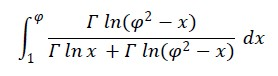Hi, all:

Thanks to all of you who posted messages and/or comments to this SRC #009 - $$\pi$$ Day 2021 Special (namely J-F Garnier, Gerson W. Barbosa, Albert Chan, PeterP, robve, Maximilian Hohmann, Ángel Martín and Massimo Gnerucci), for your interest and valuable contributions, much appreciated.

Now, these are my original results and comments for the various parts of this SRC:
• Caveat: Don't expect anything ground-breaking here, this is a simple SRC, not a full-fledged challenge or even a challenge proper, and some of you already gave the correct results and explained them thoroughly as well, so there's not much to add, this will be brief.

a.   The root of this equation in [3,4] is indeed X = $$\pi$$ ~ 3.14159265359. The base integral is: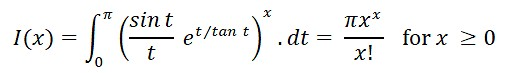so that we have:

I($$\pi$$) = $$\pi$$ $$\pi$$$$\pi$$/$$\pi$$! =
15.9359953238 ,    I(e) = $$\pi$$ ee/e! = 11.1735566407

and this simple HP-71B command-line expression will evaluate I(X) and  $$\pi$$ XX/X! for any given X ≥ 0:

>INPUT X @ INTEGRAL(0,PI,0,(SIN(IVAR)*EXP(IVAR/TAN(IVAR))/IVAR)^X);PI*X^X/GAMMA(X+1)

?1       3.14159265359      3.14159265359     {  $$\pi$$    }
?2       6.28318530717      6.2831853072      {  2 $$\pi$$  }
?EXP(1)  11.1735566407      11.1735566407     {  $$\pi$$ ee / e!  }
?PI      15.9359953238      15.9359953238     {  $$\pi$$ $$\pi$$$$\pi$$ / $$\pi$$!  }

• robve asked which quadrature procedures have I written that are better than Romberg. Well, actually several for various machines, and using them I've been able to compute definite integrals (even difficult ones) with high precision (say 100 digits and more) very fast. For the HP-71B's implementation I've achieved speeds faster than the ones achieved by the Math ROM's Romberg-based quadrature, despite the latter having the tremendous advantage of being implemented in assembly language (vs. my BASIC code) and using 15-digit/50,000-exponent precision for extended accuracy (vs. the 12-digit/499-exponent available to my BASIC code).

I'll say no more about it as my implementation is the subject matter of my article "VA036 - Boldly Going - Outsmarting INTEGRAL", to be published soon.

b.  The root of this equation in [3,4] is also X = $$\pi$$ ~ 3.14159265359. This time the base integral is: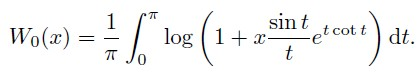which is a representation of the ubiquitous Lambert W function as a parametric definite integral, which though not new (there are other various integral representations) seems to me rather awesome nevertheless: a definite integral solves a transcendental equationW0(X) eW0(X) = X

Particularizing its value for X = 1 and exchanging W0(1) and $$\pi$$ we get: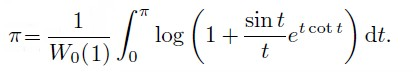from where the equation is then obtained. This HP-71B program lets us try different values of X and returns the difference between the LHS and the RHS, to see how it approaches 0 when X approaches $$\pi$$:

1  DEF FNF(T)=LN(1+SIN(T)/T*EXP(T/TAN(T)))
2  DEF FNI(X)=INTEGRAL(0,X,0,FNF(IVAR))
3  DESTROY ALL @ W=FNROOT(0,1,FVAR*EXP(FVAR)-1)
4  INPUT X @ DISP FNI(X)/W-X @ GOTO 4

[RUN]
? .1      .131342478087
? .5      .63098466783
? 1      1.1030252775
? 1.5    1.27459847697
? 2      1.08227897742
? 2.5     .6404117652
? 3       .14159265358
? 3.14    .00159265358
? 3.1415  .00009265358
? PI     -.00000000001

For X > $$\pi$$, it gives an error:  ERR L1:LOG(neg). As expected, for X = $$\pi$$ it returns ~ 0 but notice that for X ≥ 3 it returns ~ $$\pi$$ - X. Using a graphing calculator to plot the above values into a continuous graph will show why.

c.   Though this seems to be a striking finite evaluation which gives $$\pi$$ in terms of e in a much simpler and direct way than Euler's formula and without involving complex values, the magic is tarnished somewhat by the fact that e isn't really needed here, as the basic identity is:

$$\pi$$ = 4 * ( Arctan X - Arctan $$\frac{X - 1}{X + 1}$$ )

which is valid for all X > -1, as some of you explained and proved. That said, there are many interesting particular cases which you can use to trick your colleagues into believing you've discovered a brand-new, amazing evaluation for $$\pi$$. For instance:

- Using $$\pi$$ itself (!) to get $$\pi$$:  $$\pi$$ = 4 * (Arctan $$\pi$$ - Arctan $$\frac{\pi - 1}{\pi + 1}$$)

>4*(ATN(PI)-ATN((PI-1)/(PI+1))) -> 3.14159265359

- Using the Golden Ratio $$\phi$$ to get $$\pi$$:  $$\pi$$ = 4 * (Arctan $$\phi$$ - Arctan $$\frac{\phi - 1}{\phi + 1}$$)

>P=(1+SQR(5))/2 @ 4*(ATN(P)-ATN((P-1)/(P+1))) -> 3.14159265360

- Using the current year (2021) to get $$\pi$$:  $$\pi$$ = 4 * (Arctan 2021 - Arctan $$\frac{2020}{2022}$$)

>4*(ATN(2021)-ATN(2020/2022)) -> 3.14159265358

You can also trick them by using your phone number, your birthday or any number personally related to you or the person being tricked !• J-F Garnier commented that he doesn't think there's a relation which can be used to get $$\pi$$ from e but added he was thinking about finite formulae. Indeed, there are various relatively simple infinite series involving e and returning $$\pi$$ that would do fine.

He also insisted that deriving $$\pi$$ from Euler's formula as a logarithm base e (namely $$\pi$$ = loge(-1) / i ) doesn't mean that e is involved because he can use arctan instead and this is the method used to compute log(z) in various HP calculators. To this I say that the computing methods used are irrelevant, we're talking here about theoretical math, not practical implementation details, and theoretically the derivation of $$\pi$$ from Euler's formula holds and fundamentally involves e as the log base. I could give a pertinent example related to cubic equations to make it clearer but it would make this too long.

Gerson suggested replacing e by c (the speed of light in whatever units) in the formula and correctly gave the range of constants and resulting values, as well as a link to an interesting formula which, well, links e and $$\pi$$ but I don't consider that a bona-fide way to derive $$\pi$$ from e or vice versa, and the other example he gave is a definite integral, also quite unsatisfactory to me for the purpose: all sorts of integrals have $$\pi$$ as a result, thus demonstrating that $$\pi$$ is ubiquitous but nothing else. Just change $$\pi$$ to 5 and you'll see what I mean: a definite integral is no way to "derive" 5 from anything.

Last, Albert Chan and robve also explained several times and in various ways why the identity works.

d.  The summation for even dimensions from 0 to infinity of the volumes enclosed by the respective n-dimensional unit spheres (R = 1) is  e$$\pi$$ ~ 23.1406926328 (aka Gelfond's constant) and thus its $$\pi$$-th root is e, or conversely we could say that $$\pi$$ is its natural logarithm. The $$\pi$$-th root of the summation is readily obtained with the HP-71B by executing this from the command line:

>V=0 @ FOR D=0 TO 50 STEP 2 @ V=V+PI^(D/2)/FACT(D/2) @ NEXT D @ V^(1/PI)

2.71828182846

which agrees with  e ~ 2.71828182846. For the 12-digit HP-71B we stop at dimension 50 because  $$\pi$$25/25! ~ 1.73.10-13, so adding further terms won't affect the result.

Also, as I read for the first time in some Martin Gardner's book many decades ago, the volume enclosed by the n-dimensional unit sphere tends to 0 with growing n, and reaches a maximum for a (fractional) dimension between 5 (Vol = 8 $$\pi$$2/15 ~ 5.264) and 6 (Vol = $$\pi$$3/6 ~ 5.168). See if you can find this unique dimension and the corresponding maximum volume.

• Albert Chan commented that the formula gives 1 for the volume of a 0-dimensional sphere (whatever its "radius"), which seems weird to him. Well, that's by definition. Come to that, the formula also gives 2 for the volume of the 1-dimensional unit "sphere", which is but a line segment that obviously has no 3D "volume", and it also gives $$\pi$$ for the volume of the 2-dimensional unit "sphere", which is but a 2D circle with no 3D "volume" either. We tend to think of volume in terms of dimensions 3 or greater but mathematically that's not necessarily so.

e.  The song "$$\pi$$" by Kate Bush is indeed awesome, as is most of her music ("Cloudbusting", mentioned in this thread, certainly is, as is the video for it, almost a whole tragic movie told in a few minutes), and part of it appears in The Simpsons' 26th-season finale, "Mathlete's Feat", featuring about one minute of the song or so.

$$\pi$$ also appears in at least two other episodes which I watched at the time: in one of them, Prof. Frink unexpectedly says aloud that $$\pi$$ is exactly equal to 3 as a way to get some much needed attention (he sure gets it !), and in another Homer and Marge are visiting some school for "Snotty Girls and Mama's Boys" (i.e., gifted children) and two of them are singing a hand-clapping song with lyrics they've concocted to help them remember a few digits of $$\pi$$. There may be many more ... (episodes featuring $$\pi$$, that is).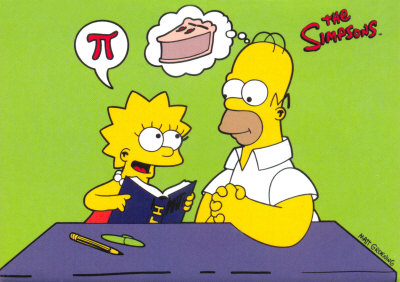• Ángel Martín briefly visited the thread to express his love for the song (and Massimo Gnerucci did likewise for the artist herself), as did Maximilian Hohmann, who also mentioned "Cloudbusting" and its pseudo-scientific background, which I knew about from reading Martin Gardner's and James Randi's books in the distant past.

f.  First, those 31,415,926,535,897 decimal places were reported by Emma Haruka Iwao on 2019's $$\pi$$ Day after 121 days of computation. Then, on January 2020 Tim Mullican computed 50 trillion digits in some 300 days, which if I'm not wrong it's the current world record as of 2021.

The resulting string of digits passes all normality tests, where we consider a real number R to be normal in some base B if any string of N base-B digits appears in the base-B representation of R with frequency B-N, e.g: in base 10 every digit 0-9 appears 1/10-th of the time, every 2-digit sequence 00-99 appears 1/100-th of the time, etc.

It can be proved that almost all real numbers are normal in any and all bases B, but rigurously proving that a "naturally-occurring" real R (i.e., not artificially defined), say $$\pi$$, is normal for even just one base B (say base 2 or 10) is excruciatingly difficult and not a single result is known so far, though the expectations are that all irrational numbers actually are, e.g. if you compute a trillion bits of $$\sqrt{2}$$, you'll find about the same number of 0's and 1's and about the same number of 00's, 01's, 10's and 11's, etc.

Not all is lost, however. If we can't (yet) prove than $$\pi$$ is normal in base 2 or 10, say, we can try to estimate the credibility of the decision "$$\pi$$ is not normal", which somewhat resembles probabilistic primality testing, where we can't rigurously prove that a certain number is prime in reasonable time but we may certify it as a probable prime if we can quickly prove that the probability of it being composite is extremely small. In the case of $$\pi$$'s normality, this has been done by analyzing the first 16 trillion bits of $$\pi$$, and the result is that the decision "$$\pi$$ is not normal" has credibility 4.3497.10-3064, which in my dictionary is akin to "impossible".

Second, the bilingual joke about "Fear of number $$\pi$$" being called "Trescatorcephobia" is a pun on 3.14 being usually said as "tres catorce" in Spanish.

Finally, re the "peer reviewed" papers demonstrating that $$\pi$$'s value is some algebraic number, I'm astonished that anyone would give them credit (let alone publish them) except for non-academic reasons. Next category: papers succinctly proving Riemann's Hypothesis or Fermat's Last Theorem in a few pages, almost casually.

• Peter P commented "where was my simple proof of Fermat's Last Theorem again? Gotta get that published lest someone steals my brilliant idea...". LOL !FLT is almost false, in the sense that there are infinitely many almost-counterexamples where the arbitrarily large LHS and RHS differ by just 1 (two other almost-counterexamples appeared in The Simpsons as well though not so close, namely 178212 + 184112 = 192212  and  398712 + 436512 = 447212). Also, a nice "flipped" variant of FLT can actually be proved using a truly "brilliant" idea.

robve gave very interesting comments and links re so-called predatory journals, as well as a related personal experience while attending an IEEE conference.

That's all for now. Again, thanks for your interest and participation in this SRC, glad you enjoyed it !

Best regards.
V.
(03-23-2021 10:20 PM)Valentin Albillo Wrote: [ -> ]Again, thanks for your interest and participation in this SRC, glad you enjoyed it !

Thank you for posting the pi day special and for the conclusion!

I moved the remainder of the long post as a separate topic on Adaptive Simpson and Romberg methods, as suggested: https://www.hpmuseum.org/forum/thread-16523.html

- Rob
b. answer to what this program computes:

10 P=1
20 FOR I=2 TO 1000 STEP 2
30 P=P*I/(I-1)
40 DISP 2*P*P/(I+1)
50 NEXT I

As Albert correctly points out and explains in his reply, the program computes Wallis Product.

$$\frac{\pi}{2} = \prod_{n=1}^\infty \frac{4n^2}{4n^2-1} = \frac{2\cdot 2\cdot 4\cdot 4\cdot 6\cdot 6\cdot 8\cdot 8\cdots}{1\cdot 3\cdot 3\cdot 5\cdot 5\cdot 7\cdot 7\cdot 9\cdots}$$

The proof is "typically featured in modern calculus textbooks" according to this Wikipedia article.

Another way to express the Wallis Product in compact form with factorials:

$$\frac{\pi}{2} \approx \begin{cases} \displaystyle\frac{n\,(n-1)!!^2}{n!!^2} & \text{if n\ge 3 is odd} \\ \displaystyle\frac{n!!^2}{(n+1)\,(n-1)!!^2} & \text{if n\ge 2 is even} \end{cases}$$

where $$n!!$$ is the double factorial.

Of course, we would never want our programs to use such factorials in the numerator and denominator, because of overflow and loss of floating point precision.

There are many ways this product can be implemented in code. The code shown above instantiates this product's numerator directly as 2*P*P to make this relation of this code to the product form more readily apparent.

Wallis product very slowly converges to $$\pi$$, much slower than Viète's formula and other hiistorical infinite series formulas for $$\pi$$. It is not a practical algorithm to compute $$\pi$$ to many decimals.

Efficient algorithms exist to compute $$\pi$$ in many decimals, such as the following short C program (160 characters when compacted) written by Dik T. Winter at CWI (Centrum voor Wiskunde en Informatica - the national research institute for mathematics and computer science) Amsterdam to compute $$\pi$$ up to 800 decimal digits:

int a = 10000, b, c = 2800, d, e, f, g;
main() {
for (; b-c; ) f[ b++] = a/5;
for (; d=0, g = c*2; c-=14, printf("%.4d", e+d/a), e = d % a)
for (b = c; d += f[ b]*a, f[ b] = d % --g, d /= g--, --b; d *= b)
;
}

31415926535897932384626433832795028841971693993751058209749445923078164062862089​98628034825342117067982148086513282306647093844609550582231725359408128481117450​28410270193852110555964462294895493038196442881097566593344612847564823378678316​52712019091456485669234603486104543266482133936072602491412737245870066063155881​74881520920962829254091715364367892590360011330530548820466521384146951941511609​43305727036575959195309218611738193261179310511854807446237996274956735188575272​48912279381830119491298336733624406566430860213949463952247371907021798609437027​70539217176293176752384674818467669405132000568127145263560827785771342757789609​17363717872146844090122495343014654958537105079227968925892354201995611212902196​08640344181598136297747713099605187072113499999983729780499510597317328160963185​

Other programs to compute the Wallis Product

main = putStrLn (show (wallis 1000)) where
wallis n = (2*p^2/(n+1)) where
(_,p) = until done next (n,1.0)
next (k,p) = (k-2,p*k/(k-1))
done (k,p) = (k==0)

C (imperative PL):

int main() {
int i, n = 1000; // must be even
double p = 1;
for (i = 2; i <= n; i += 2)
p *= (double)i/(i-1);
printf("%g\n", 2*p*p/(n-1));
}

Prolog (logic PL):

wallis(W) :- prod(1000, P), W is 2*P*P/1001.
prod(0, 1) :- !.
prod(K, Q) :- M is K-2, prod(M, P), Q is P*K/(K-1).

- Rob
Pages: 1 2 3
Reference URL's
• HP Forums: https://www.hpmuseum.org/forum/index.php
• :